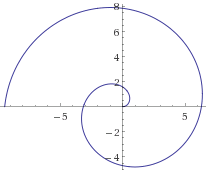Algebra

# Parametric Equations Problem Solving

The line segment connecting two points $(-5, 9)$ and $(3, -2)$ can be expressed in parametric equations as $x=-a+bt, y=c-dt. \quad (0 \leq t \leq 1),$

where $t=0$ corresponds to the point $(-5, 9)$. What is the value of $a+b+c+d$?

Let $a\pi$ be the area of the region bounded by the curve defined by the parametric equations $x=7\cos 3t, \quad y=7\sin 3t,$ where $0 \leq t \leq 2\pi$. What is the value of $a$?

If $(a, 0)$ is the intersection point of the positive part of the $x$-axis and the curve defined by the parametric equations $x=\sin 4t, y=6\cos 8t \quad (0 \leq t \leq 2\pi),$ what is the value of $\frac{1}{a^4}?$.What is the polar equation of this graph?

Two clowns, Twinkle and Jingle, are throwing pies at each other. Twinkle throws a pie toward Jingle from $500$ centimeters away. Its flight path is given by parametric equations $\begin{cases} x &=& 100t \\ y &=& 80t - 16t^2 \end{cases}$ where $t$ is time in seconds.

Two seconds later Jingle launches an interceptor pie from his location with the flight path $\begin{cases} x &=& 500 - 500(t-2) \\ y &=& K(t-2) - 16(t-2)^2 \end{cases}$ Find the value of $K$ which will guarantee that the interceptor pie will hit its target (the pie thrown by Twinkle).

×